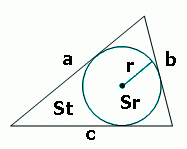# Triangle Incircle Calculator: Radius, Area, and Ratio

The Triangle Incircle Calculator is a tool that allows you to determine the properties of the incircle of a triangle based on its side lengths. By entering the lengths of the three sides, this calculator calculates the radius and area of the incircle, which is the largest circle that can fit inside the triangle.Since the incircle is described by its radius, you only need to find it using the formula R = S / p, where R represents the radius, S is the triangle's area, and p is the triangle's semiperimeter.

In addition to the incircle properties, the calculator also provides the area of the triangle itself. This allows you to compare the size of the incircle with the overall triangle area. The calculator further computes the ratio between the incircle area and the triangle area, giving you a quantitative measure of how much of the triangle is filled by the incircle.#### Incircle of a triangle

Digits after the decimal point: 2